Updating search results...

# 1532 Results

View
Selected filters:
• EngageNY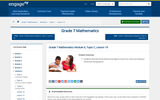Conditional Remix & Share Permitted
CC BY-NC-SA
Rating
0.0 stars
Overview:

In this lesson, students describe three-dimensional figures built from cubes by looking at horizontal slicing planes.

Subject:
Mathematics
Material Type:
Lesson Plan
Provider:
EngageNY
02/09/2017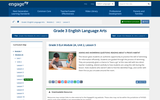Conditional Remix & Share Permitted
CC BY-NC-SA
Rating
3.0 stars
Overview:

In this lesson, students continue to build their reading skills by asking questions and reading sections of the text, Everything You Need to Know about Frogs and Other Slippery Creatures. Students continue to build their general knowledge about frogs by reading selections about life cycle, skin, and habitat.

Subject:
English Language Arts
Material Type:
Lesson Plan
Provider:
EngageNY
04/19/2017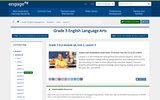Conditional Remix & Share Permitted
CC BY-NC-SA
Rating
2.0 stars
Overview:

In this lesson, students build their reading skills by asking questions and reading sections of the text, Everything You Need to Know about Frogs and Other Slippery Creatures. Students build their general knowledge about frogs by reading selections about life cycle, skin, and habitat.

Subject:
English Language Arts
Material Type:
Lesson Plan
Provider:
EngageNY
04/19/2017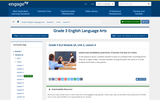Conditional Remix & Share Permitted
CC BY-NC-SA
Rating
5.0 stars
Overview:

In this lesson, students continue to build their reading skills by asking questions and reading sections of the text, Everything You Need to Know about Frogs and Other Slippery Creatures. Students continue to build their general knowledge about frogs by reading selections about life cycle, skin, and habitat.

Subject:
English Language Arts
Material Type:
Lesson Plan
Provider:
EngageNY
04/19/2017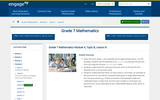Conditional Remix & Share Permitted
CC BY-NC-SA
Rating
0.0 stars
Overview:

Given the exact value, x, of a quantity and an approximate value, a, of the quantity, students use the absolute error, | a - x |, to compute the percent error by using the formula | a - x |/ |x| x 100%.
Students understand the meaning of percent error as the percent the absolute error is of the exact value.
Students understand that when an exact value is not known, an estimate of the percent error can still be computed when given a range determined by two inclusive values (e.g., if there are known to be between 6,000 and 7,000 black bears in New York, but the exact number is not known, the percent error can be estimated to be (1,000/6,000)(100%) at most, which is 16(2/3)%).

Subject:
Mathematics
Material Type:
Lesson Plan
Provider:
EngageNY
01/30/2017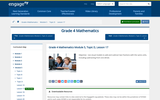Conditional Remix & Share Permitted
CC BY-NC-SA
Rating
0.0 stars
Overview:

For this lesson, students use visual models to add and subtract two fractions with the same units including subtracting from one whole.

Subject:
Mathematics
Material Type:
Lesson Plan
Provider:
EngageNY
02/24/2017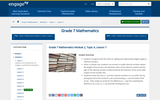Conditional Remix & Share Permitted
CC BY-NC-SA
Rating
0.0 stars
Overview:

In this lesson, students recognize that the rules for adding and subtracting integers apply to rational numbers. Given a number line, students use arrows to model rational numbers where the length of the arrow is the absolute value of the rational number and the sign of the rational number is determined by the direction of the arrow with respect to the number line. Students locate the sum p + q of two rational numbers on a number line by placing the tail of the arrow for q at p and locating p + q at the head of the arrow. They create an arrow for the difference p - q by first rewriting the difference as a sum, p + (- q), and then locating the sum.

Subject:
Mathematics
Material Type:
Lesson Plan
Provider:
EngageNY
01/24/2017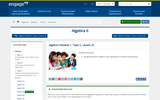Rating
0.0 stars
Overview:

Students perform addition and subtraction of rational expressions.

Subject:
Mathematics
Material Type:
Lesson Plan
Provider:
EngageNY
02/02/2018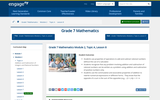Conditional Remix & Share Permitted
CC BY-NC-SA
Rating
0.0 stars
Overview:

In this lesson, students use properties of operations to add and subtract rational numbers without the use of a calculator. Students recognize that any problem involving addition and subtraction of rational numbers can be written as a problem using addition and subtraction of positive numbers only. Students use the commutative and associative properties of addition to rewrite numerical expressions in different forms. They know that the opposite of a sum is the sum of the opposites (e.g., - (3 + (-4)) = -3 + 4.

Subject:
Mathematics
Material Type:
Lesson Plan
Provider:
EngageNY
01/24/2017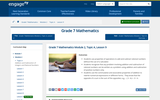Conditional Remix & Share Permitted
CC BY-NC-SA
Rating
0.0 stars
Overview:

In this lesson, students use properties of operations to add and subtract rational numbers without the use of a calculator. Students recognize that any problem involving addition and subtraction of rational numbers can be written as a problem using addition and subtraction of positive numbers only. Students use the commutative and associative properties of addition to rewrite numerical expressions in different forms. They know that the opposite of a sum is the sum of the opposites; e.g., - ( 3 - 4) = -3 + 4.

Subject:
Mathematics
Material Type:
Lesson Plan
Provider:
EngageNY
01/24/2017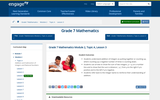Conditional Remix & Share Permitted
CC BY-NC-SA
Rating
4.0 stars
Overview:

In this lesson, students understand addition of integers as putting together or counting up, where counting up a negative number of times is counting down. Students use arrows to show the sum of two integers, p + q, on a number line and to show that the sum is distance | q | from p to the right if q is positive and to the left if q is negative. Students refer back to the Integer Game to reinforce their understanding of addition.

Subject:
Mathematics
Material Type:
Lesson Plan
Provider:
EngageNY
01/24/2017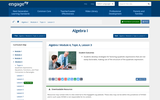Rating
0.0 stars
Overview:

Lesson with application of factoring quadratic expressions and word problems.

Subject:
Mathematics
Material Type:
Lesson Plan
Provider:
EngageNY
04/02/2018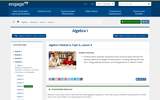Rating
0.0 stars
Overview:

Lesson showing how to factor quadratics specifically when leading coefficient is not 1.

Subject:
Mathematics
Material Type:
Lesson Plan
Provider:
EngageNY
04/02/2018Rating
0.0 stars
Overview:

In this lesson, students rewrite simple rational expressions in different forms, including representing remainders when dividing.

Subject:
Mathematics
Material Type:
Lesson Plan
Provider:
EngageNY
07/27/2018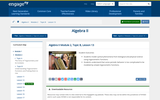Rating
0.0 stars
Overview:

In this lesson, students model cyclical phenomena from biological and physical science using trigonometric functions. Students understand that some periodic behavior is too complicated to be modeled by simple trigonometric functions.

Subject:
Mathematics
Material Type:
Lesson Plan
Provider:
EngageNY
08/15/2018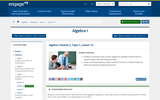Rating
0.0 stars
Overview:

In this lesson, students summarize data on two categorical variables collected from a sample using a two-way frequency table. Given a two-way frequency table, students construct a relative frequency table and interpret relative frequencies.

Subject:
Mathematics
Material Type:
Lesson Plan
Provider:
EngageNY
08/15/2018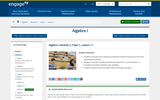Rating
0.0 stars
Overview:

In this lesson, students summarize data on two categorical variables collected from a sample using a two-way frequency table. Given a two-way frequency table, students construct a relative frequency table and interpret relative frequencies.

Subject:
Mathematics
Material Type:
Lesson Plan
Provider:
EngageNY
08/15/2018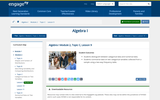Rating
0.0 stars
Overview:

In this lesson, students distinguish between categorical data and numerical data. Students summarize data on two categorical variables collected from a sample using a two-way frequency table.

Subject:
Mathematics
Material Type:
Lesson Plan
Provider:
EngageNY
08/15/2018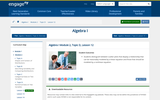Rating
4.0 stars
Overview:

In this lesson, students distinguish between scatter plots that display a relationship that can be reasonably modeled by a linear equation and those that should be modeled by a nonlinear equation.

Subject:
Mathematics
Material Type:
Lesson Plan
Provider:
EngageNY
08/15/2018Rating
4.0 stars
Overview:

In this lesson, students distinguish between scatter plots that display a relationship that can be reasonably modeled by a linear equation and those that should be modeled by a nonlinear equation. Students use an equation given as a model for a nonlinear relationship to answer questions based on an understanding of the specific equation and the context of the data.

Subject:
Mathematics
Material Type:
Lesson Plan
Provider:
EngageNY
08/15/2018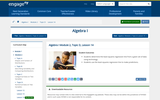Rating
0.0 stars
Overview:

In this lesson, students determine the least-squares regression line from a given set of data using technology. Students use the least-squares regression line to make predictions.

Subject:
Mathematics
Material Type:
Lesson Plan
Provider:
EngageNY
08/13/2018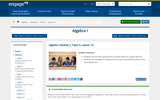Rating
4.0 stars
Overview:

In this lesson, students use the least-squares line to predict values for a given data set. Students use residuals to evaluate the accuracy of predictions based on the least-squares line.

Subject:
Mathematics
Material Type:
Lesson Plan
Provider:
EngageNY
08/13/2018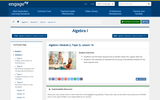Rating
4.0 stars
Overview:

In this lesson, students use the least-squares line to predict values for a given data set. Students use residuals to evaluate the accuracy of predictions based on the least-squares line.

Subject:
Mathematics
Material Type:
Lesson Plan
Provider:
EngageNY
08/13/2018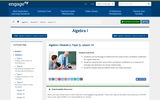Rating
0.0 stars
Overview:

In this lesson, students use technology to determine the value of the correlation coefficient for a given data set. Students interpret the value of the correlation coefficient as a measure of strength and direction of a linear relationship. Students explain why correlation does not imply causation.

Subject:
Mathematics
Material Type:
Lesson Plan
Provider:
EngageNY
08/15/2018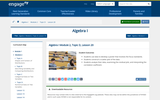Rating
0.0 stars
Overview:

In this lesson, students use data to develop a poster that involves the focus standards. Students construct a scatter plot of the data. Students analyze their data, examining the residual plot, and interpreting the correlation coefficient.

Subject:
Mathematics
Material Type:
Lesson Plan
Provider:
EngageNY
08/15/2018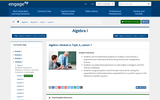Rating
0.0 stars
Overview:

In this lesson, students use the distributive property to multiply a monomial by a polynomial and understand that factoring reverses the multiplication process. Students use polynomial expressions as side lengths of polygons and find area by multiplying. Students recognize patterns and formulate shortcuts for writing the expanded form of binomials whose expanded form is a perfect square or the difference of perfect squares.

Subject:
Mathematics
Material Type:
Lesson Plan
Provider:
EngageNY
08/13/2018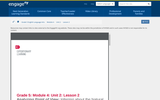Conditional Remix & Share Permitted
CC BY-NC-SA
Rating
4.0 stars
Overview:

Students describe how a narrator's point of view influences the description of events.

Subject:
English Language Arts
Material Type:
Lesson Plan
Provider:
EngageNY
Author:
Engage NY
04/23/2014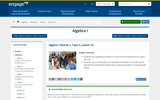Rating
0.0 stars
Overview:

Lesson on creating and analyzing residual graphs.

Subject:
Mathematics
Material Type:
Lesson Plan
Provider:
EngageNY
04/02/2018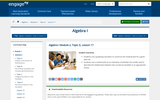Rating
0.0 stars
Overview:

Lesson and practice on analyzing graphs with residuals.

Subject:
Mathematics
Material Type:
Lesson Plan
Provider:
EngageNY
04/02/2018Rating
0.0 stars
Overview:

Students make sense of a contextual situation that can be modeled with a linear, quadratic, or exponential function when presented as a word problem. They analyze a verbal description and create a model using an equation, graph, or table.

Subject:
Mathematics
Material Type:
Lesson Plan
Provider:
EngageNY
03/30/2018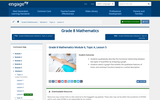Conditional Remix & Share Permitted
CC BY-NC-SA
Rating
0.0 stars
Overview:

In this lesson, students qualitatively describe the functional relationship between two types of quantities by analyzing a graph. Students sketch a graph that exhibits the qualitative features of linear and nonlinear functions based on a verbal description.

Subject:
Mathematics
Material Type:
Lesson Plan
Provider:
EngageNY
02/23/2017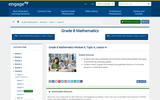Conditional Remix & Share Permitted
CC BY-NC-SA
Rating
0.0 stars
Overview:

In this lesson, students describe qualitatively the functional relationship between two types of quantities by analyzing a graph. Students sketch a graph that exhibits the qualitative features of a function based on a verbal description.

Subject:
Mathematics
Material Type:
Lesson Plan
Provider:
EngageNY
02/21/2017Rating
0.0 stars
Overview:

Students will prove the angle-angle criterion for two triangles to be similar and use it to solve triangle problems.

Subject:
Mathematics
Material Type:
Lesson Plan
Provider:
EngageNY
02/01/2018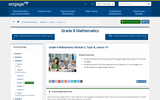Conditional Remix & Share Permitted
CC BY-NC-SA
Rating
0.0 stars
Overview:

In this lesson, students know an informal proof of the Angle-Angle (AA) criterion for similar triangles. Students present informal arguments as to whether or not triangles are similar based on Angle-Angle criterion.

Subject:
Mathematics
Material Type:
Lesson Plan
Provider:
EngageNY
02/16/2017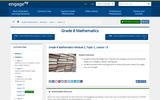Conditional Remix & Share Permitted
CC BY-NC-SA
Rating
0.0 stars
Overview:

In this lesson, students know the Angle Sum Theorem for triangles; the sum of the interior angles of a triangle is always 180 degrees. Students present informal arguments to draw conclusions about the angle sum of a triangle.

Subject:
Mathematics
Material Type:
Lesson Plan
Provider:
EngageNY
02/16/2017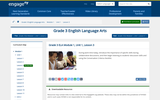Only Sharing Permitted
CC BY-NC-ND
Rating
0.0 stars
Overview:

This lesson introduces students answer questions using specific details from the text and explain what they understand about the topic being discussed.

Subject:
English Language Arts
Material Type:
Lesson Plan
Provider:
EngageNY
04/13/2017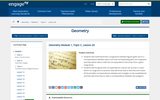Rating
0.0 stars
Overview:

Students understand that a congruence between figures gives rise to a correspondence between parts such that corresponding parts are congruent, and they are able to state the correspondence that arises from a given congruence.

Subject:
Mathematics
Material Type:
Lesson Plan
Provider:
EngageNY
03/30/2018Rating
0.0 stars
Overview:

Students use systems of equations or inequalities to solve contextual problems and interpret solutions within a particular context.

Subject:
Mathematics
Material Type:
Lesson Plan
Provider:
EngageNY
02/05/2018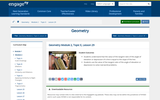Rating
0.0 stars
Overview:

Students understand that the value of the tangent ratio of the angle of elevation or depression of a line is equal to the slope of the line. Students use the value of the tangent ratio of the angle of elevation or depression to solve real-world problems.

Subject:
Mathematics
Material Type:
Lesson Plan
Provider:
EngageNY
02/02/2018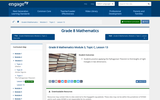Conditional Remix & Share Permitted
CC BY-NC-SA
Rating
0.0 stars
Overview:

In this lesson, students practice applying the Pythagorean Theorem to find lengths of right triangles in two dimensions.

Subject:
Mathematics
Material Type:
Lesson Plan
Provider:
EngageNY